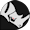# Using Rhino to Solve Mathematical Equations

Rhino is a 3-D modeling application that can solve graphically. For example, if you want to know if two curves or surfaces intersect, you can use the Intersect command. If you want to know the centroid, volume or principal moments, you can ask Rhino that, too.

You can also use Rhino to solve mathematical equations. But Rhino does not have commands like solve equation or plot graph. The standard keyboard is also a problem. Where is the square root sign, superscript, or subscript?

So, you will need to use scripting. Rhino has a script editor made specifically to help with these problems.

Rhino 5 contains two scripting languages:

Rhino 4 contains only RhinoScript.

Because of this, we recommend using RhinoScript.Ask questions on our discourse support forum. When posting questions, please use either the Rhino Developer or the Scripting category.

## The basics

To illustrate how you can use RhinoScript to solve mathematical problems, I have written two simple scripts that solve the first two questions of the math practice test.

Note, both of these solutions can be copied and pasted into the RhinoScript editor (EditScript) for testing. You also need to know the commands LoadScript and RunScript.

The basic steps are:

1. Write a script function in script editor EditScript.
2. Or load a an existing file with the .rvb extension into the script editor.
4. Use the RunScript command to run the function name.

Note: Dragging a .rvb file onto the Rhino window will load and run the script.

• From the Rhino Help menu, click Plug-ins and then click RhinoScript.

Also see VB Fundamentals.

## Examples

### 1. Graph the following equation: y = (x - 4)^2 = 2

```' Define range of x values
xrange = Array(-4, -2, 0, 2, 4, 6, 8, 10)
' With each x value
For Each x In xrange
' Solve for y
y = (x-4)^2 + 2
' Add a point at the x,y location
Next```

## 2. Equation f(x) = x^2 - 5x - 14

Find zeros of function using these values: 2, 7, -2, -7, then print to Rhino command prompt.

```' Define range of x values
xrange = Array(2, 7, -2, -7)
' With each x value
For Each x In xrange
' Solve for y, where y = f(x)
y = (x^2) - (5*x) - 14
' If y is zero, print x
If y = 0 Then Rhino.Print x
Next```